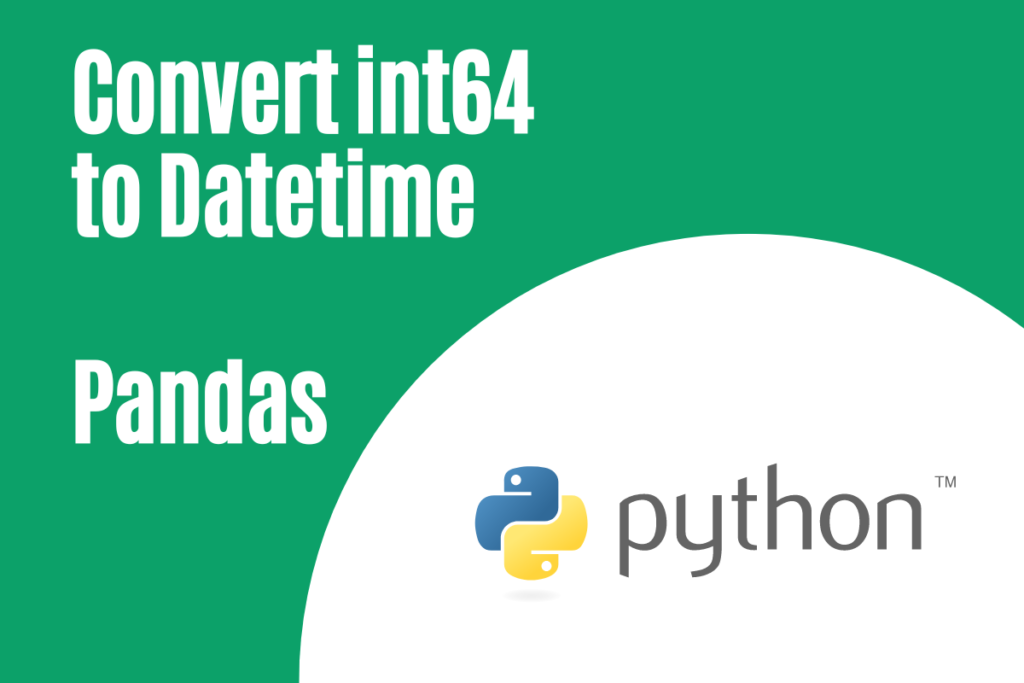# How to Convert int64 to Datetime in Pandas?When dealing with datasets, they need not necessarily be in the format that we require. At times, even numerical data can be stored in textual format and instances like those might throw tantrums at the pace at which we progress, especially when gargantuan amounts of data are involved. Is there a way around this? Can anything in Python help to put us out of this misery? This article attempts to provide answers to these questions by demonstrating solutions for a specific case, one where we convert numbers into date & time format.

We shall look into the conversion of int64 data into Datetime format for each of the following categories.

• Converting long dates
• Converting short dates
• Converting dates with time

To convert int64 to Datetime in Pandas, use the pd.to_datetime() function. For long dates (YYYYMMDD), use the format ‘%Y%m%d’. For short dates (YYMMDDD), use ‘%y%m%d’. For dates with time (YYMMDDHHMM), use ‘%y%m%d%H%M’. Adjust the format parameter to suit your specific date representation.

The to_datetime( ) function from the pandas library would be used for converting the int64 data into datetime. So, let us get started by importing the pandas library using the below code.

```import pandas as pd
```

## Converting Long Dates (YYYYMMDD)

This category deals with the dates that are stored in the form of continuous numbers in the int64 format. Let us have a close look at what appears when the following dataset is printed using the print function.

```df = pd.DataFrame({'When?':[20200316, 20230517, 20210525, 20221219, 20191130],
'What?': ['Convocation', 'Exams End', 'Vacation starts', 'Tour', 'Party']})
print(df)
print(df.dtype)
```

It is evident from the above image that the data type is int64 and it is obvious that the data is not in any conventional means by which a date could be represented. So, it is time to take things in hand and do something about it using the to_datetime( ) function.

```df['When?'] = pd.to_datetime(df['When?'],format = '%Y%m%d')
```

Run the above code and try printing the dataframe again using the print function. Also, have a look at the data types in the resulting dataframe, you might be in for a surprise.

It is such a relief to see the dates in the format they are supposed to be, isn’t it?

## Converting Short Dates (YYMMDD)

Sometimes the dates in the numerical format can also be in their short forms taking only the two digits of the year rather than four. This might pose a problem when one deploys the technique detailed in the above section to convert those values into datetime format. So, here is what needs to be done, a minor tweak in the code and we are all set.

Better to witness a demonstration rather than talk it out, so have a look at the below dataset.

```df = pd.DataFrame({'When?':[200316, 230517, 210525, 221219, 191130],
'What?': ['Convocation', 'Exams End', 'Vacation starts', ‘Tour’, ‘Party’]})
print(df)
print(df.dtypes)
```

The minor tweak here is to replace ‘%Y’ with ‘%y’ in the to_datetime function as shown below.

```df['When?'] = pd.to_datetime(df['When?'],format = '%y%m%d')
```

Now run the code & print the results to witness the changes in the date format for all the values under the ‘When?’ column.

## Converting Dates with Time (YYMMDDHHMM)

There can also be instances when the date is combined with the time and listed together. It might sound like a herculean task to sieve the dates from time, but it ain’t the case with Python. Let us have a look at how to resolve this in the following example.

```df = pd.DataFrame({'When?':[2003161915, 2305171300, 2105250700, 2212191030,
1911302130], 'What?': ['Convocation', 'Exams End',
'Vacation starts','Tour', 'Party']})
print(df)
print(df.dtypes)
```

The last four digits in each value under the ‘When?’ column corresponds to the time in 24-hour format. Let us now separate it out from the date alongside converting the numerical value into a date using the to_datetime( ) function. Include ‘%H%M’ to isolate the time from the date.

```df['When?'] = pd.to_datetime(df['When?'],format = '%y%m%d%H%M')
```

Run the above code and the results emerge when the print function is used to return the modified dataframe.